# Unity游戏图形渲染效果系列之阴影篇(二)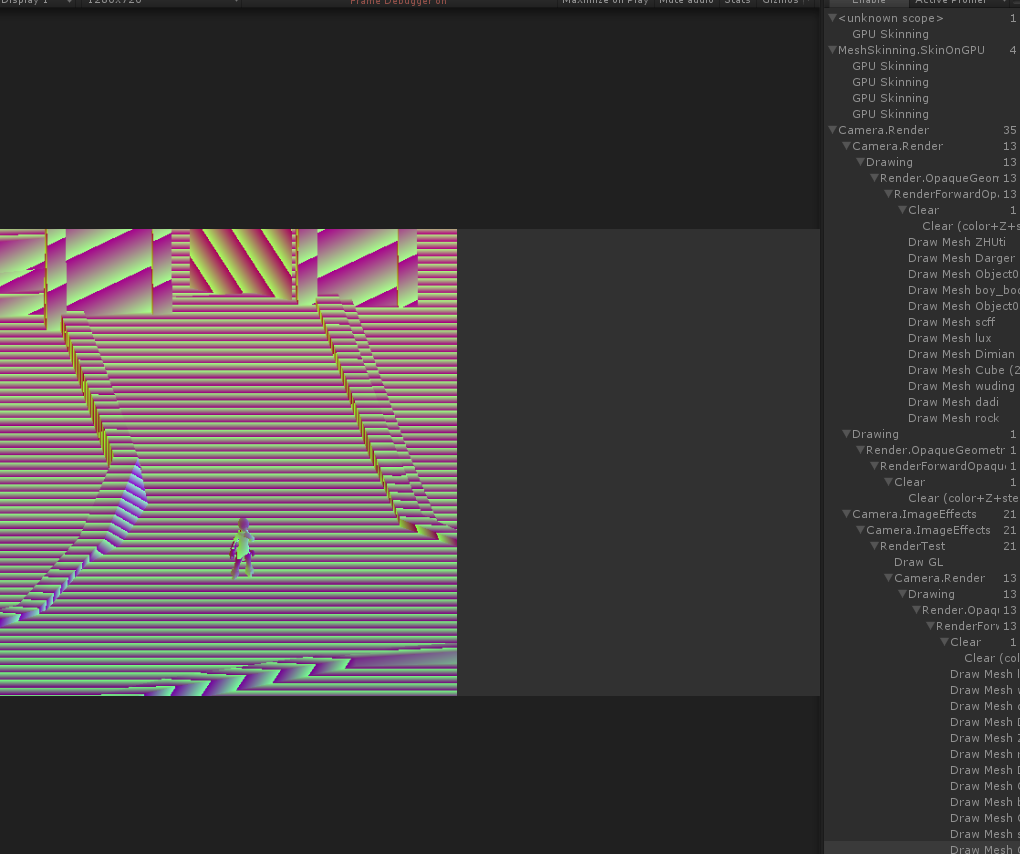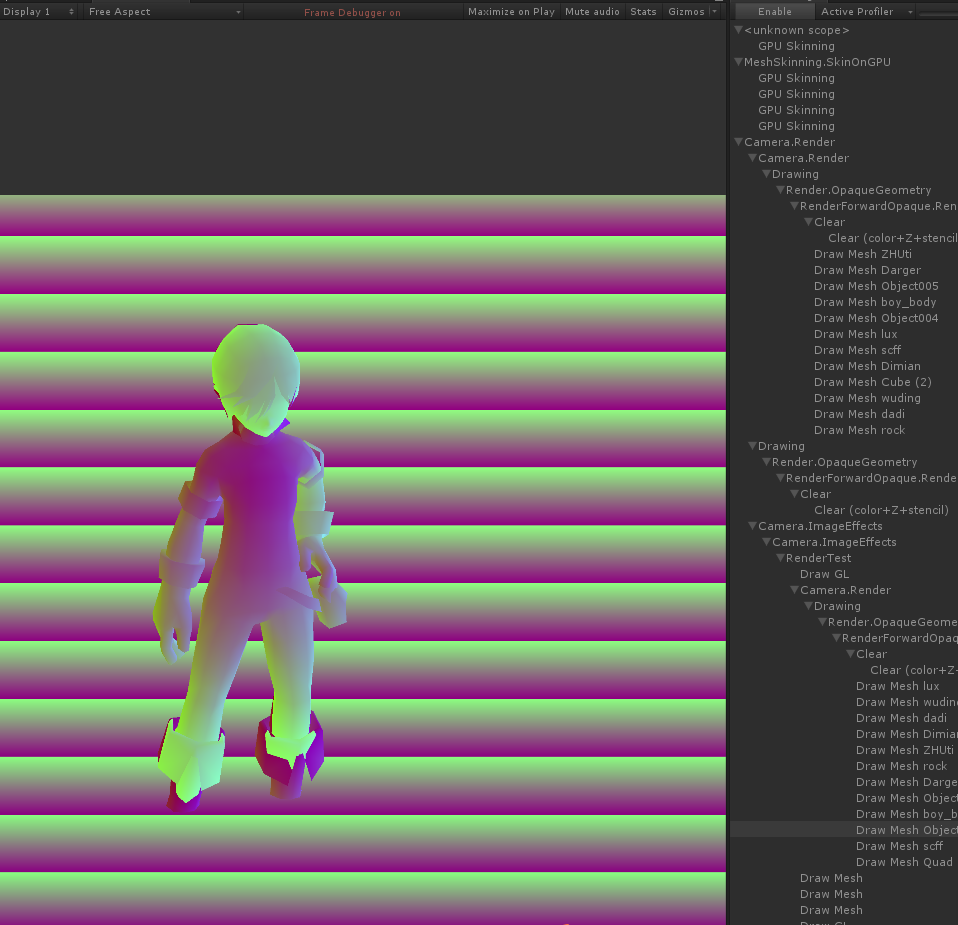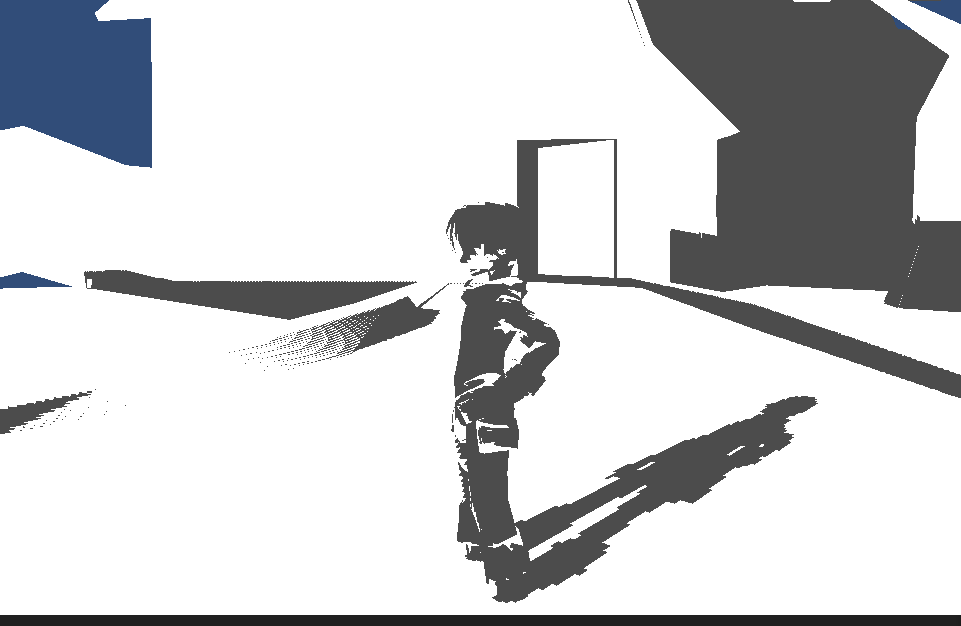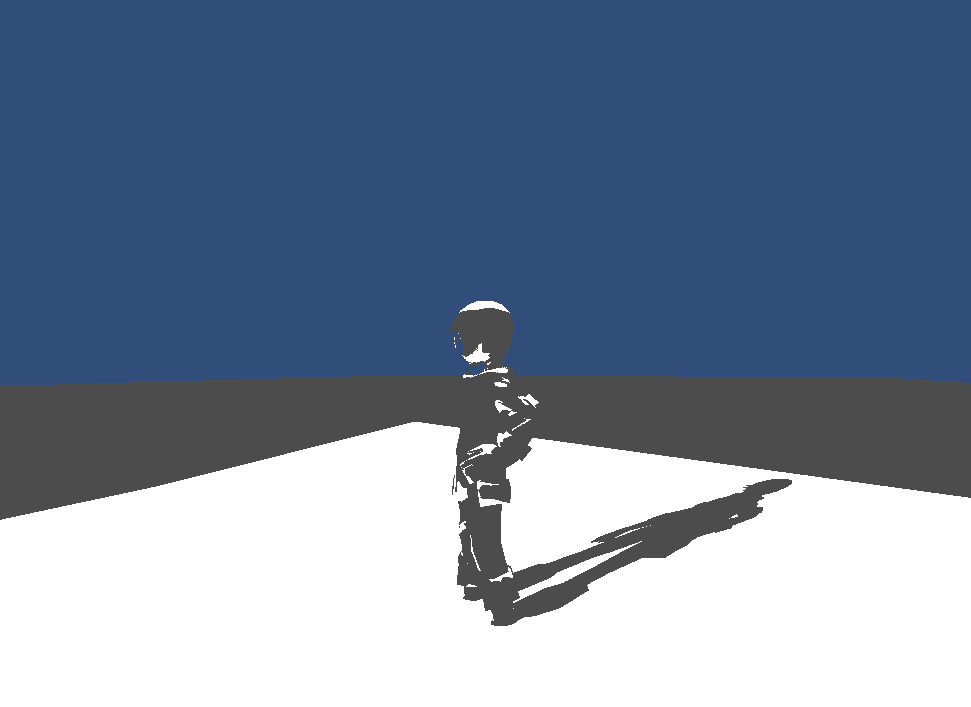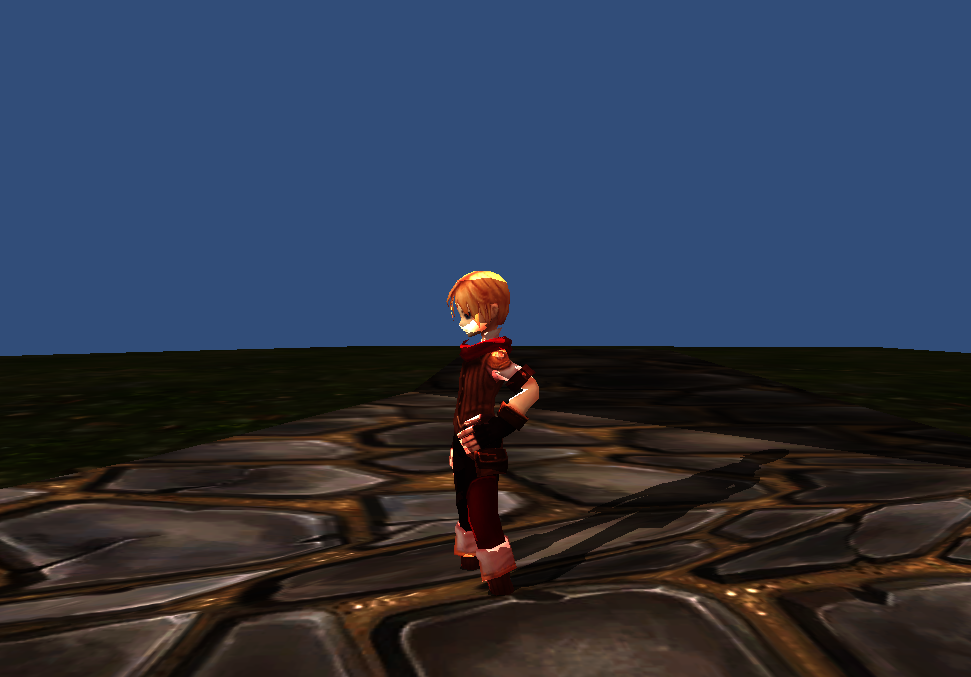1级：基础阴影，可能只对深度贴图采样一次参与计算，性能高，但是效果很差。

2级：中级阴影，对深度贴图进行2-4次采样参与计算，通过在y方向lerp的方式，会对阴影效果有一点点软化效果。

3级：高级阴影或者叫软阴影，对深度贴图进行9次以上的采样和lerp，效果可以达到很好的效果了。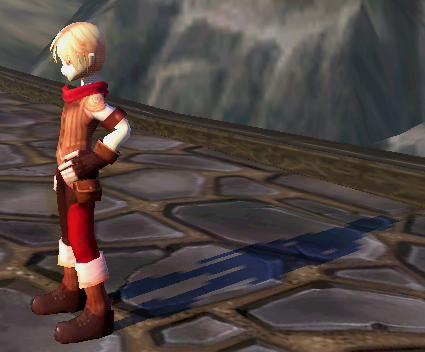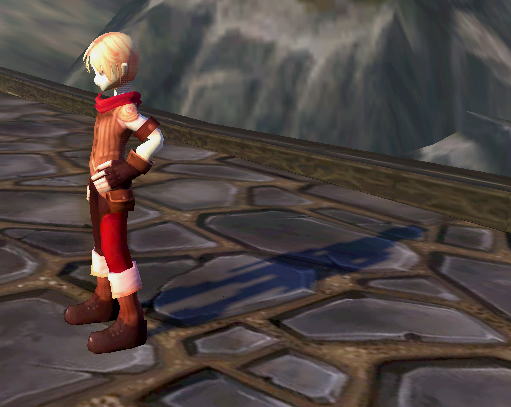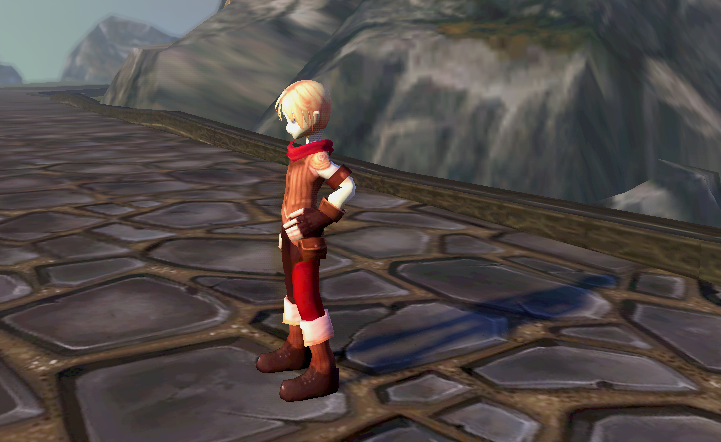在第一章的文章中，我们实现了最简单的阴影系数的求解方法，如下：

	float4 col = tex2D(_ShadowDepth, depuv);
float olddepth = DecodeDepth(col.xy);//0-1
float newdepth = i.shadowvpos.z  / invShadowViewport.w;//farclip
float occ=newdepth-olddepth;
		return occ;//其中的ooc就是阴影系数，要么是0，要么是1，就把阴影和非阴影的区域分开了。

	float occ=0;
float Num=9;
for (int i = 0; i < Num; i++)//这里的Num可以根据自己调整可以是4 也可以是9，更好的效果 16或者20都行。
{
float2 temp = uv+offsetuv[i]*0.01f;
float2 temp2 = uv+offsetuv[i+1]*0.02f;
float4 col = tex2D(_ShadowDepth, temp);
float4 col2 = tex2D(_ShadowDepth, temp2);
float olddepth = DecodeDepth(col.xy);
float olddepth2 = DecodeDepth(col2.xy);
float dot_val.x=olddepth-olddepth2>0;
.
.
.
float2 lerp_val = frac((uv+invVP.xy)*1024.0f);
float2 temp = lerp(dot_val.xy, dot_val.zw, lerp_val.y);
occ+= lerp(temp.x, temp.y, lerp_val.x);
}

return occ/Num;

float2 offsetuv = {float2(1,0),float2(1,-1),float2(0,1),float2(1,1),
float2(-1,0),float2(-1,1),float2(0,-1),float2(-1,-1),
float2(-1,0),float2(-1,1),float2(0,-1),float2(-1,-1)};
float newdepth = z/ invShadowViewport.w;
float old_depth = DecodeDepth(tex2D(_ShadowDepth, uv).xy);
float dis = newdepth-old_depth;
if(dis<0)
{
return 1;//非阴影点 就不需要采样了
}

float occ =0;
float sss=0.001;
float pix=0.002;
for (int i = 0; i < 12; i++)//这里的Num可以根据自己调整可以是4 也可以是9，更好的效果 16或者20都行。
{
float depth0 = DecodeDepth(tex2D(_ShadowDepth, uv+offsetuv[i]*pix).xy);
if(abs(old_depth-depth0)>sss)
occ+=0.1;

}
return 0.5+occ;